# Rationality theorems

(diff) ← Older revision | Latest revision (diff) | Newer revision → (diff)

for algebraic groups

Statements about the rationality (unirationality) or non-rationality of various algebraic group varieties (cf. Rational variety, Unirational variety). Since Abelian varieties can never be rational, the main interest is in rationality theorems for linear algebraic groups. Here, the rationality problem has two essentially different features: geometrical and arithmetical, according as whether the ground fieldis algebraically closed or not. The first rationality theorems over the fieldof complex numbers were in fact proved by E. Picard and, in contemporary terminology, establish the unirationality of varieties of connected complex groups. The rationality problem for group varieties was clearly stated by C. Chevalley  as late as 1954. Progress in this direction is closely connected with achievements in the structure theory of algebraic groups. Thus, the Levi decomposition enables one to reduce the rationality problem to the case of reductive groups, and the Bruhat decomposition is the key to proving the rationality of varieties of reductive groups over any algebraicallyclosed field (cf. Reductive group). Thus, in the geometrical case definitive results have been obtained.

The situation for algebraically non-closed fieldsturns out to be much more complicated. Examples of non-rational-varieties are supplied by algebraic tori; for example, a three-dimensional torus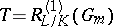, corresponding to the biquadratic extension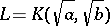of(see ). This example is minimal, for tori of dimension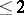are rational. Algebraic tori are always unirational. Arbitrary connected-groups are not necessarily unirational , but ifis perfect oris reductive, unirationality can be proved (see ). Thus, the rationality problem for group varieties has the character of the Lüroth problem over an algebraically non-closed field.

Since an arbitrary reductive group is the almost-direct product of a torus and a semi-simple group, one can naturally distinguish two main cases: 1)is a torus; or 2)is a semi-simple group. The first case is investigated using various cohomological invariants (for semi-simple groups, these invariants turn out to be ineffective). Fairly complete results are obtained for tori which split over an Abelian extension of the ground field (see ). The first example of a non-rational variety in the class of semi-simple groups was a non-simply-connected group, whose construction is actually contained in . The resulting conjecture, that varieties of simply-connected groups are always rational, was solved negatively by V.P. Platonov, using reduced-theory (see , ). It was found that the reduced Whitehead group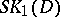of a finite-dimensional central simple-algebrais trivial if the variety determined by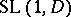is rational over. These results carry over to unitary groups . There are results on the rationality of the spinor varieties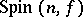, whereis a non-degenerate quadratic form invariables over(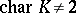). Spinor varieties are rational if either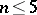, oris locally compact and non-discrete, or is the field of rational numbers (see , , ); for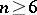there are spinor varieties that are not rational . The last result is astonishing, in thatis a two-sheeted covering of the rational variety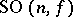.

The term "rationality theorem" is sometimes used in the theory of algebraic groups in a somewhat different sense, in connection with assertions about the properties of groups over a, not necessarily algebraically closed, field, such as the Rosenlicht–Grothendieck theorem, which states that any connected-group possesses a maximal torus defined over(see ).

How to Cite This Entry:
Rationality theorems. Encyclopedia of Mathematics. URL: http://encyclopediaofmath.org/index.php?title=Rationality_theorems&oldid=18791
This article was adapted from an original article by A.S. Rapinchuk (originator), which appeared in Encyclopedia of Mathematics - ISBN 1402006098. See original article